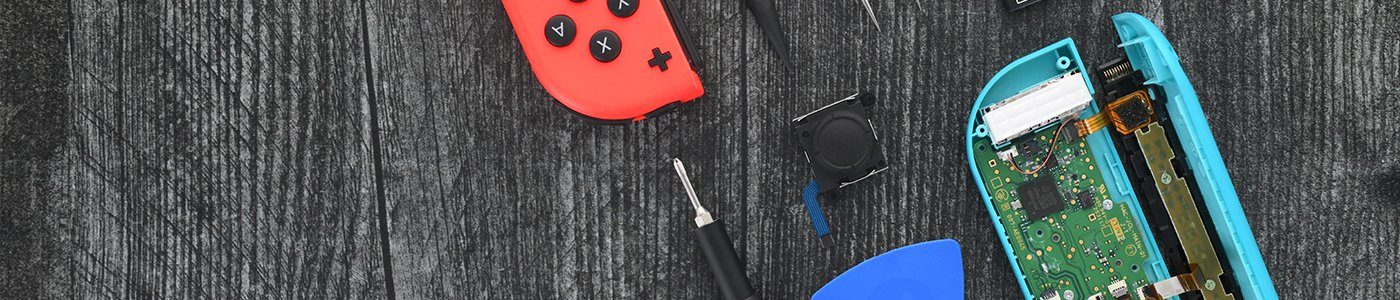跳转到主内容

53 个问题 查看全部

# Why does my multimeter measure different voltages?

So I have a multimeter that whenever I swap out the conductor, the voltage increases/decreases even though my battery is the same, such as when I swap out a wire for water or a wire of different length

EDIT: I'm trying to measure voltage, however the voltage changes depending on what the conductor is. It's set to VDC but whenever I swap out the conductor the voltage reading changes

andretran41 either add an image or draw a schematic of how , where and what you are measuring. How many volts from your power source? what conductors? How are you changing those? What voltage scale is your meter set at? What make is your meter? Use this guide 在已经存在的问题里加入图片 to add images. That way we can see what you see and we can try to understand your setup.### 任天堂 Switch 套装

#### 快速恢复到游戏的状态## 1个回答

Hi,

What are you trying to measure?

Have you used a multimeter before?

If not, have you read the User manual?

It sounds as though you are measuring resistance (in Ohms) not voltage.

You won't read any voltage in water to easily.

Check what measuring scale the meter is set to. Is it VDC or VAC or Ohms? What is the make and model number of the meter that you are using?

The resistance reading will vary depending on the conductivity of the material that you have connected between the meter's test leads.

I'm trying to measure voltage, however the voltage changes depending on what the conductor is. It's set to VDC but whenever I swap out the conductor the voltage reading changes.

Hi,

To measure voltage you need a voltage source. What conductor do you mean?

Normally you connect the meter's test leads across 2 points in the circuit to get a reading e.g. between the +ve part of the circuit and the -ve part as normally a power supply is connected this way.

If you are trying to measure the voltage in series with a conductor it won't work (that is how you measure current) and measuring voltage between two points along a conductor will vary because all you are measuring is the potential difference (PD or voltage difference) if there is any, between those two points, not the voltage with reference to earth (which is usually the negative side of the power supply)

The voltage drop along a conductor will vary depending on its resistance and the current flowing through it due to the applied voltage

Ohm's Law

E = IR where E -Voltage, I- current, R- resistance

So I've created a circuit using a 12V battery, and I've taken one of the ends and connected it to the test probe, with the other one I've connected it to a conductor and connected that to the other test probe. I'm fairly new to all this so I'm not sure if I'm doing it correctly or not. EDIT: I'm trying to measure the voltage after it goes through the conductor.

Hi,

Doing the way you described you have your meter in series with the conductor. Voltmeters are never used in series. They measure in parallel to the circuit.

Connect the conductor to the circuit you created.

Place your meter test leads between the "output" side of the conductor (other end of conductor away from the circuit you created. and the -ve side of the battery.

This reading should be what you want.

As I'm not quite sure what you mean by "created a circuit", place your meter test leads between the "input" to the conductor (coming from the circuit you created) and the -ve side of the battery and measure the voltage, just in case it is not the same as the supply voltage because of the "circuit you created".

As has been mentioned, draw a picture of your setup it makes it so much easier.

## 添加你的答案andretran41 将永远感激不已